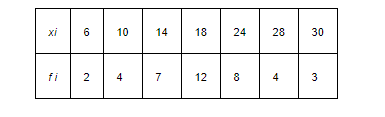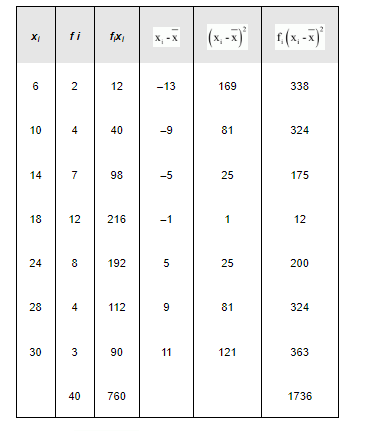# Find the mean and variance for the data

Question:

Find the mean and variance for the dataSolution:

The data is obtained in tabular form as follows.Here, $N=40, \sum_{i=1}^{T} f_{i} x_{i}=760$

$\therefore \bar{x}=\frac{\sum_{i=1}^{7} f_{i} x_{i}}{N}=\frac{760}{40}=19$

Variance $=\left(\sigma^{2}\right)=\frac{1}{\mathrm{~N}} \sum_{i=1}^{7} \mathrm{f}_{i}\left(\mathrm{x}_{\mathrm{i}}-\overline{\mathrm{x}}\right)^{2}=\frac{1}{40} \times 1736=43.4$